# The voltage across a 100-μF capacitor takes thefollowing values. Calculate the expression for thecurrent through the capacitor in each case.a. vC(t) = 40 cos(20t − π/2) Vb. vC(t) = 20 sin 100t Vc. vC(t) = −60 sin(80t + π/6) Vd. vC(t) = 30 cos(100t + π/4) V

Question
14 views

The voltage across a 100-μF capacitor takes the
following values. Calculate the expression for the
current through the capacitor in each case.
a. vC(t) = 40 cos(20t − π/2) V
b. vC(t) = 20 sin 100t V
c. vC(t) = −60 sin(80t + π/6) V
d. vC(t) = 30 cos(100t + π/4) V

check_circle

Step 1

Given capacitor voltage and value of capacitor is –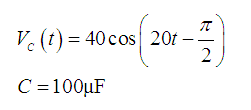Value of current is –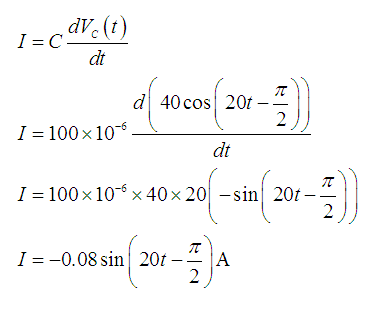Step 2

Given capacitor voltage and value of capacitor is –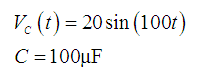Value of current is –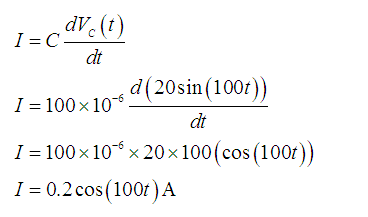Step 3

Given capacitor voltage and value of capacitor is –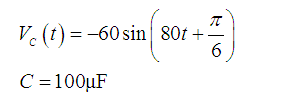Value of current is –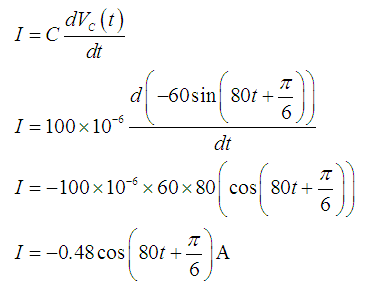...

### Want to see the full answer?

See Solution

#### Want to see this answer and more?

Solutions are written by subject experts who are available 24/7. Questions are typically answered within 1 hour.*

See Solution
*Response times may vary by subject and question.
Tagged in